### An Introduction to the Kalman Filter

Kalman Filters are a form of predictor-corrector used extensively in control systems engineering for estimating unmeasured states of a process. The estimated states may then be used as part of a strategy for control law design.

This tutorial discusses the original Kalman Filter formulation which was developed for linear discrete-time processes (or at least processes that may be modeled with sufficient accuracy as a linear discrete-time process). Other tutorials discuss non-linear forms of the Kalman Filter -- the Extended Kalman Filter and the Unscented Kalman Filter -- and a continuous time formulation -- the Kalman-Bucy Filter.Figure 1: Linear discrete-time process with input and measurement noise.

Consider the linear discrete-time process shown in Figure 1 which may be written in the standard state-space form,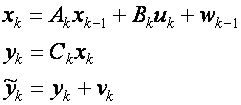Here

• k denotes a discrete point in time (with k-1 being the immediate past time point).
• uk is a vector of inputs
• xk is a vector of the actual states, which may be observable but not measured.
• yk is a vector of the actual process outputs.
•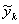is a vector of the measured process outputs.
• wk and vk are process and output noise respectively. They are assumed to be zero mean Gaussian with covariance Qk and Rk respectively.

Given the inputs, measured outputs and assumptions on the process and output noise, the purpose of the Kalman Filter is to estimate unmeasured states (assuming they are observable) and the actual process outputs. This is shown in Figure 2 where the estimated states are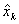, andare the estimated measured outputs.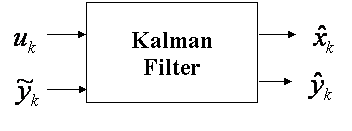Figure 2: Input-output of the Kalman Filter.

The Kalman filter uses a 2 step predictor-corrector algorithm. The first step involves projecting both the most recent state estimate and an estimate of the error covariance (from the previous time period) forwards in time to compute a predicted (or a-priori) estimate of the states at the current time. The second step involves correcting the predicted state estimate calculated in the first step by incorporating the most recent process measurement to generate an updated (or a-posteriori) state estimate.

Mathematically, the predictor step is given by,And the corrector step is given by,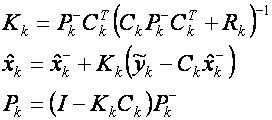In the above equations Pk is an estimate of the covariance of the measurement error and Kk is called the Kalman gain. After both the prediction and correction steps have been performed thenis the current estimate of the states andcan be calculated directly from it. Bothand Pk are stored and used in the predictor step of the next time period.

A simple example demonstrating how to implement a Kalman filter in Simulink can be found here.